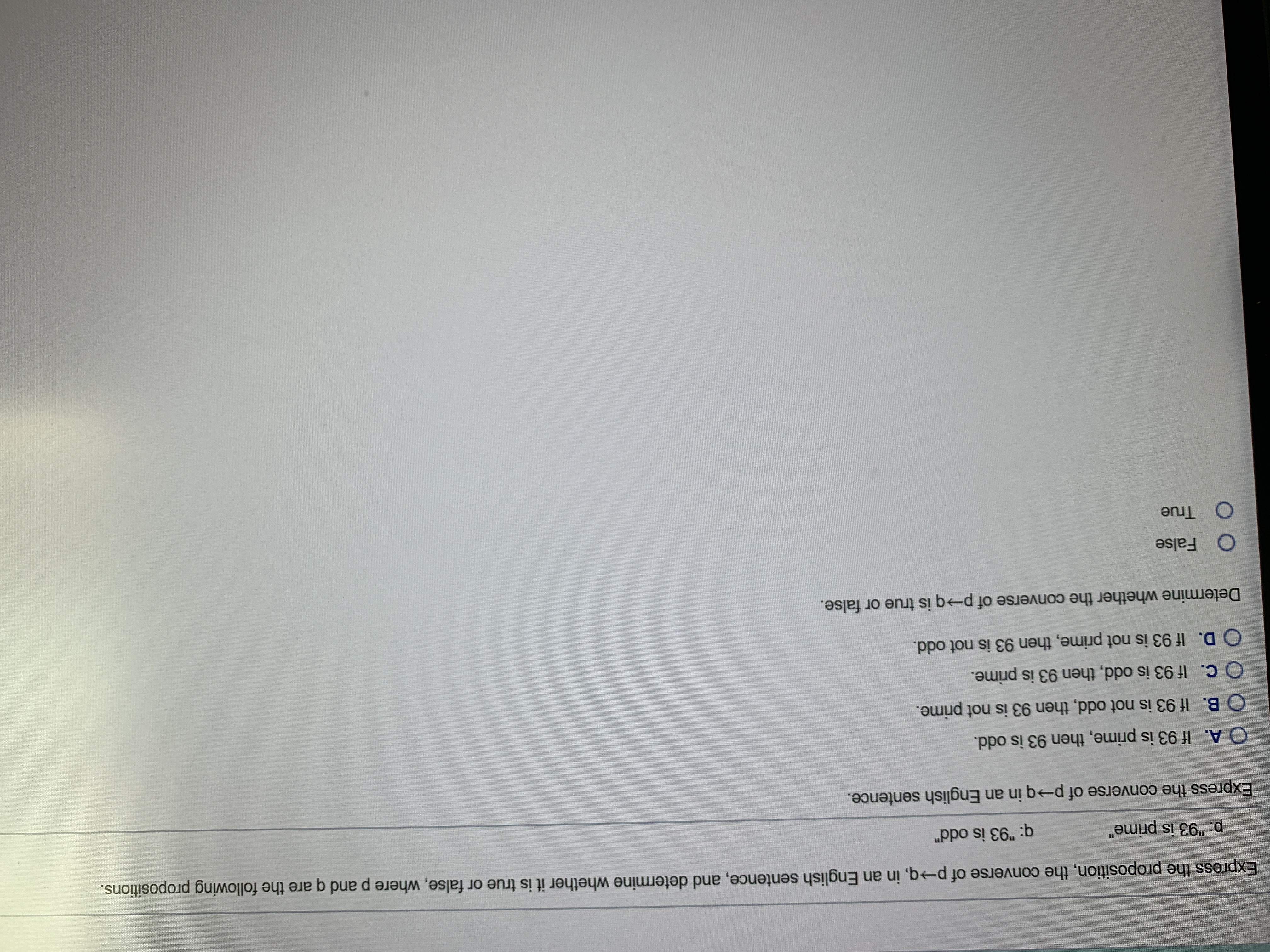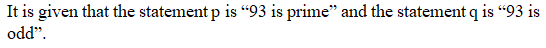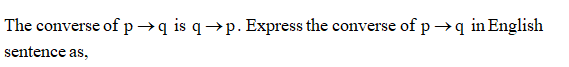# Express the proposition, the converse of p→q, in an English sentence, and determine whether it is true or false, where p and q are the following propositions.p: "93 is prime"q: "93 is odd"Express the converse of p→q in an English sentence.O A. If 93 is prime, then 93 is odd.B. If 93 is not odd, then 93 is not prime.O C. If 93 is odd, then 93 is prime.O D. If 93 is not prime, then 93 is not odd.Determine whether the converse of pq is true or false.FalseTrue

Question
4 viewshelp_outlineImage TranscriptioncloseExpress the proposition, the converse of p→q, in an English sentence, and determine whether it is true or false, where p and q are the following propositions. p: "93 is prime" q: "93 is odd" Express the converse of p→q in an English sentence. O A. If 93 is prime, then 93 is odd. B. If 93 is not odd, then 93 is not prime. O C. If 93 is odd, then 93 is prime. O D. If 93 is not prime, then 93 is not odd. Determine whether the converse of p q is true or false. False True fullscreen
check_circle

Step 1Step 2Step 3

Therefore, the correct op...

### Want to see the full answer?

See Solution

#### Want to see this answer and more?

Solutions are written by subject experts who are available 24/7. Questions are typically answered within 1 hour.*

See Solution
*Response times may vary by subject and question.
Tagged in

### Math